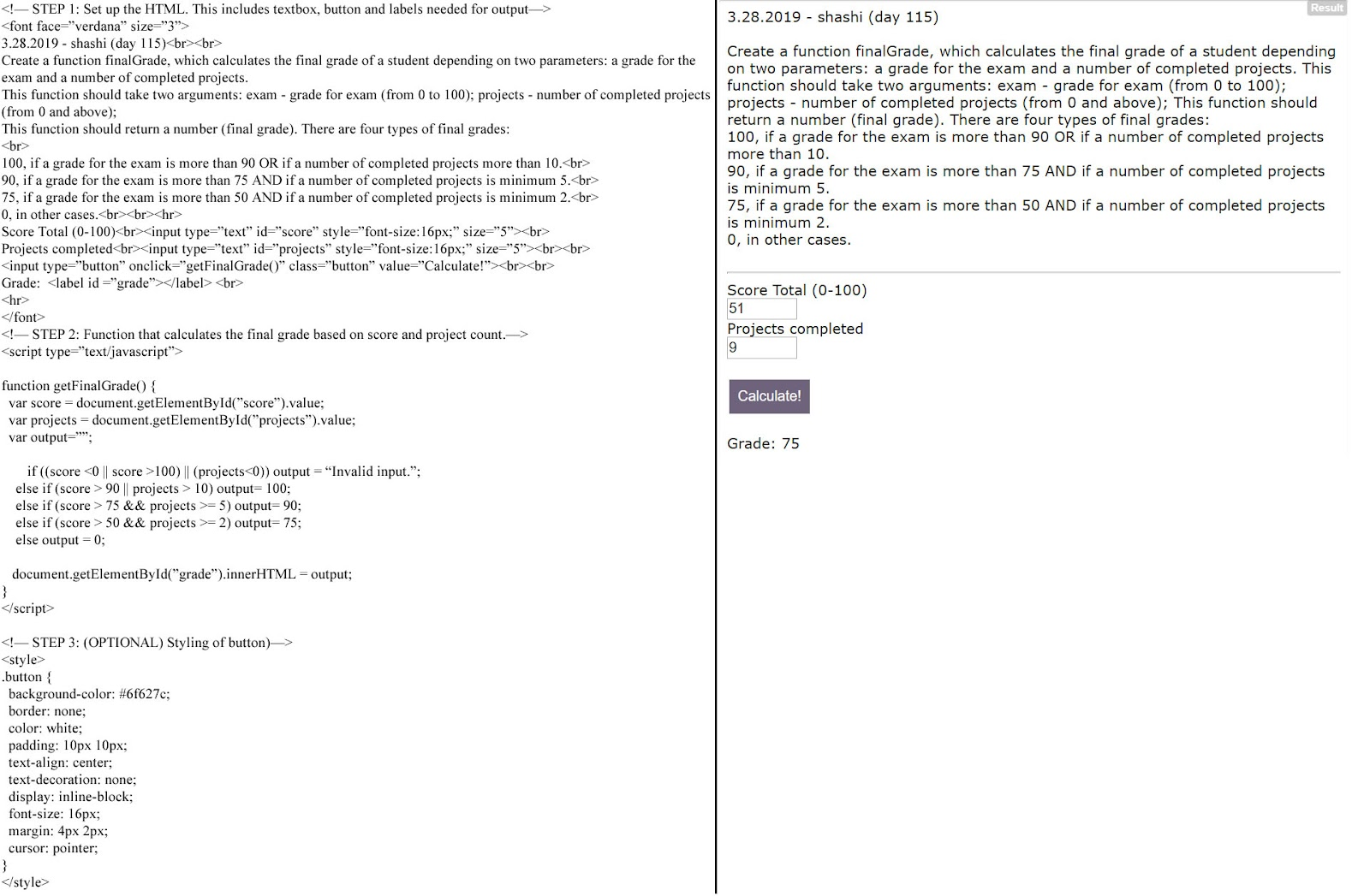3.1.2019 | Accept 2 arrays and print items that exist (even partially) in both arrays.

3.2.2019 | Accept 2 strings, get the sum of their ASCII values and check if the two match.

3.3.2019 | Accept a name and return a nickname version of it

3.4.2019 | Accept recipe + available ingredients and return how many cakes can be baked.

3.5.2019 | Accept a sentence and reverse words > 3 characters.

3.6.2019 | Movie ticket purchase simulation using arrays and loops

3.7.2019 | Town’s population calculator depending on growth percentage.

3.8.2019 | Accept String array of words and format a sentence from them.

3.9.2019 - Morse Code Decoder

3.10.2019 - Add two numbers without using the + operator (Bitwise operators)

3.11.2019 - Cipher/Encryption program that converts first letter to ASCII and swaps 2nd and last letter.

3.12.2019 - Accept a number and return a String separated/grouped by appearance of even/odd digits.

3.13.2019 - Stack based post fix notation processor.

3.14.2019 | Accept text and format it to specified width

3.15.2019 | Accept String and create an extended, increasing version of itself.

3.16.2019 | Accept number and return number of times it need to multiply within for a single digit to result.

3.17.2019 | Accept column number and return relevant Excel/Google sheet column heading.

3.18.2019 | Check if a readable string exists in a scrambled string pattern.

3.19.2019 | Accept mirror image of a clock time and return the actual time.

3.20.2019 | Greed game dice roll calculator

3.21.2019 | Pizza order rewarding system calculator

3.22.2019 | Simple sentence reverser in Java

3.23.2019 | Accept string and treat # symbol as a backspace

3.24.2019 | Check if a number can be produced by adding any 2 numbers in array.

3.25.2019 | Bowling pin processor that removes pins based on numbers.

3.26.2019 | Program accepts truck driver schedule and prints whether or not overtime is reached.

3.27.2019 | Accept a number, convert to Binary and swap its even/odd bits.

3.28.2019 | Accept score (0-100) and project completion count to print a final grade.

### 3.2.2019 | Accept 2 strings, get the sum of their ASCII values and check if the two match.

 #3.2.2019 - shashi# program that accepts 2 strings and finds the sum of their ASCII values. If they match, TRUE returned. Else, FALSE.def compareASCIIsum(string1,string2): #function starts here        #STEP 1: Error check validation    if not string1 or not string2:        return "Invalid inputs"    #STEP 2: Get the sum of a valid String 1 and String 2    sumOfString1 = 0    if string1 is not '':        for char in string1.upper():    #we will convert all characters to UPPERCASE for consistency            sumOfString1 = sumOfString1 + ord(char)    sumOfString2 = 0    if string2 is not '':        for char in string2.upper():            sumOfString2 = sumOfString2 + ord(char)    #STEP 3: Compare the 2 values. If they are equal, return TRUE.    if sumOfString1 == sumOfString2:        return True        #ELSE: return FALSE.        return False#end of function#testing functionsprint(compareASCIIsum("AD","BC"))      #A (65) + D (68) =   133 and B(66) + C(67) = 133. So they are equal. (TRUE)print(compareASCIIsum("AC","DD"))       #A (65) + C (67) =  132  and D (68) + D (68) = 136. Not equal.       (FALSE)print(compareASCIIsum("ZzZz", "ffPFF")) #ZZZZ  = 90x4 = 360   AND ffPFF (70x4 + 80 = 280 + 80 = 360). Both are equal. (TRUE)print(compareASCIIsum("", "FG"))            #blank string sent. "Invalid inputs" returned. (ERROR)print(compareASCIIsum("FG", ""))            #blank string sent. "Invalid inputs" returned. (ERROR)#end of program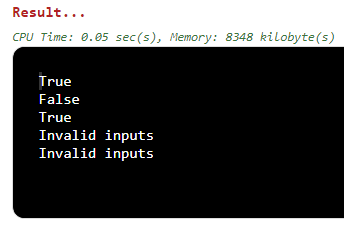### 3.3.2019 | Accept a name and return a nickname version of it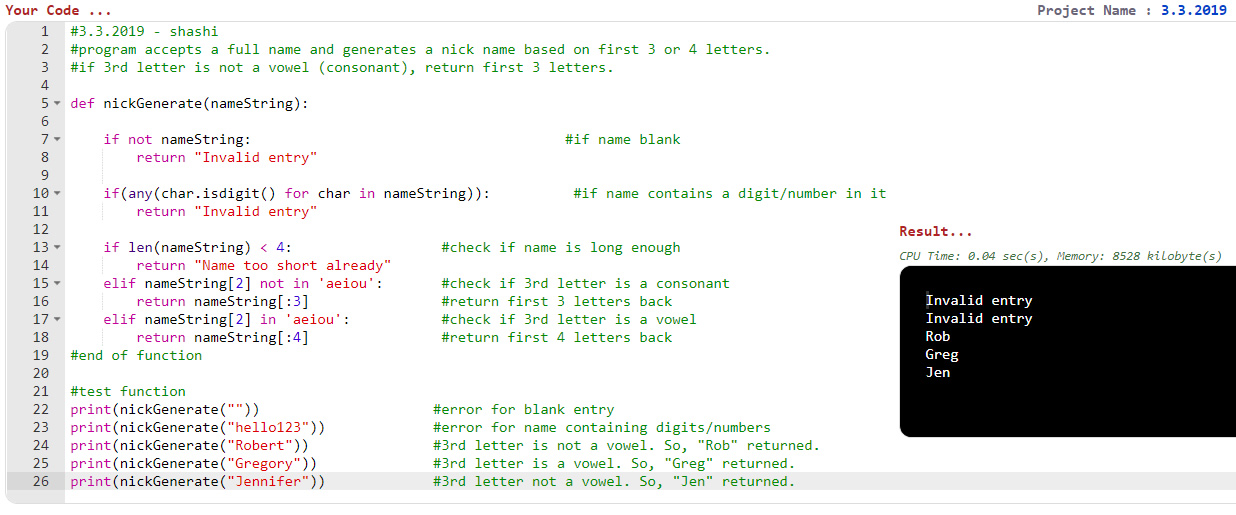### 3.4.2019 | Accept recipe + available ingredients and return how many cakes can be baked.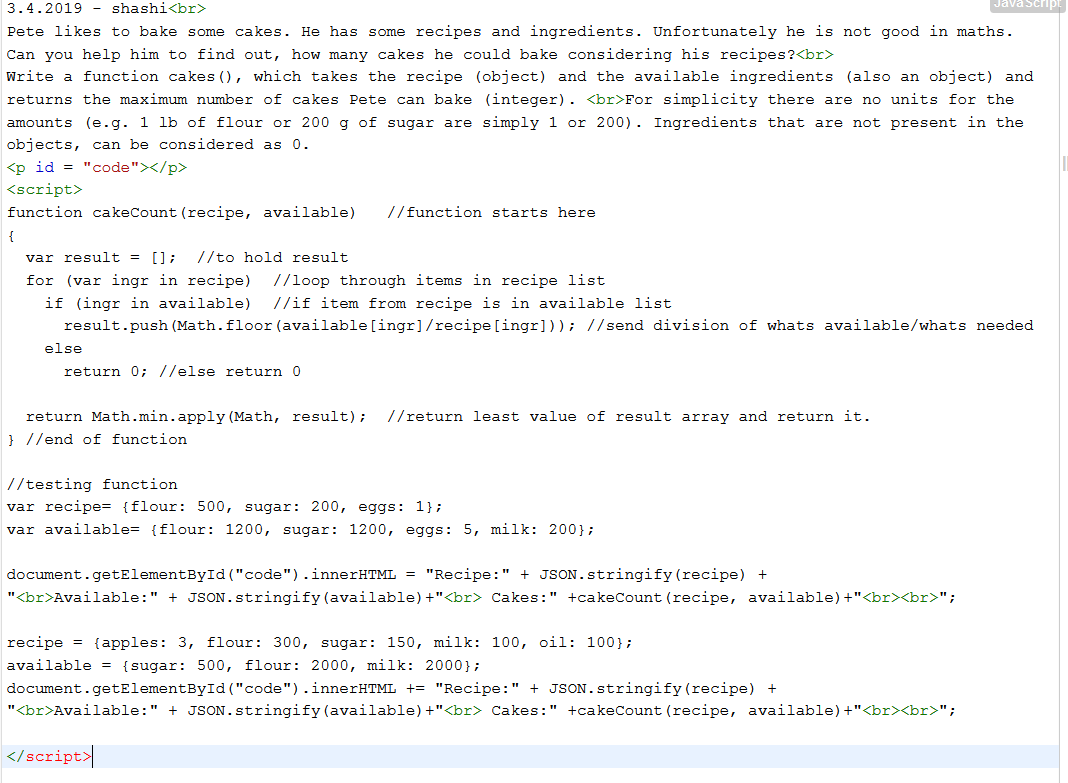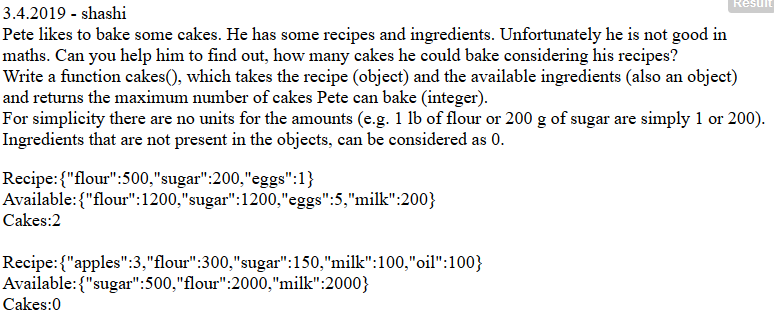### 3.5.2019 | Accept a sentence and reverse words > 3 characters.

 //3.5.2019 - shashi //program that accepts a sentence and reverses all words that are >3 characters. import java.util.Arrays; //import util.Arrays to implement array functions public class rotateSentence {//class begins here public static void main(String[] args) //main() begins here    System.out.println(rotateWords("Hello! This is a test!")); //function call    System.out.println(rotateWords("She sells sea shells on the sea shore.")); //function call }//end of main()//function starts here public static String rotateWords(String sentence){   String[] words = sentence.split(" "); //split words in the String into an array   for (int i=0; i 3) { //if word length is >3, then reverse it.           words[i] = new StringBuilder(words[i]).reverse().toString(); //replace same element with reversed version of it.         } //end if     }//end loop return String.join(" ",words); //return joined version of array with a space delimiter. }//function ends here }//class ends here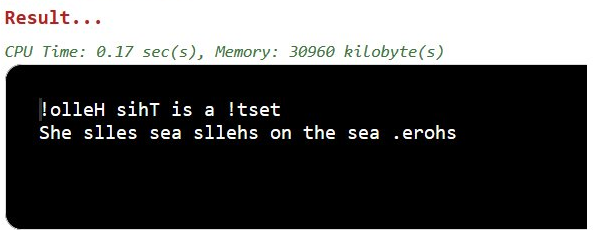### 3.6.2019 | Movie ticket purchase simulation using arrays and loops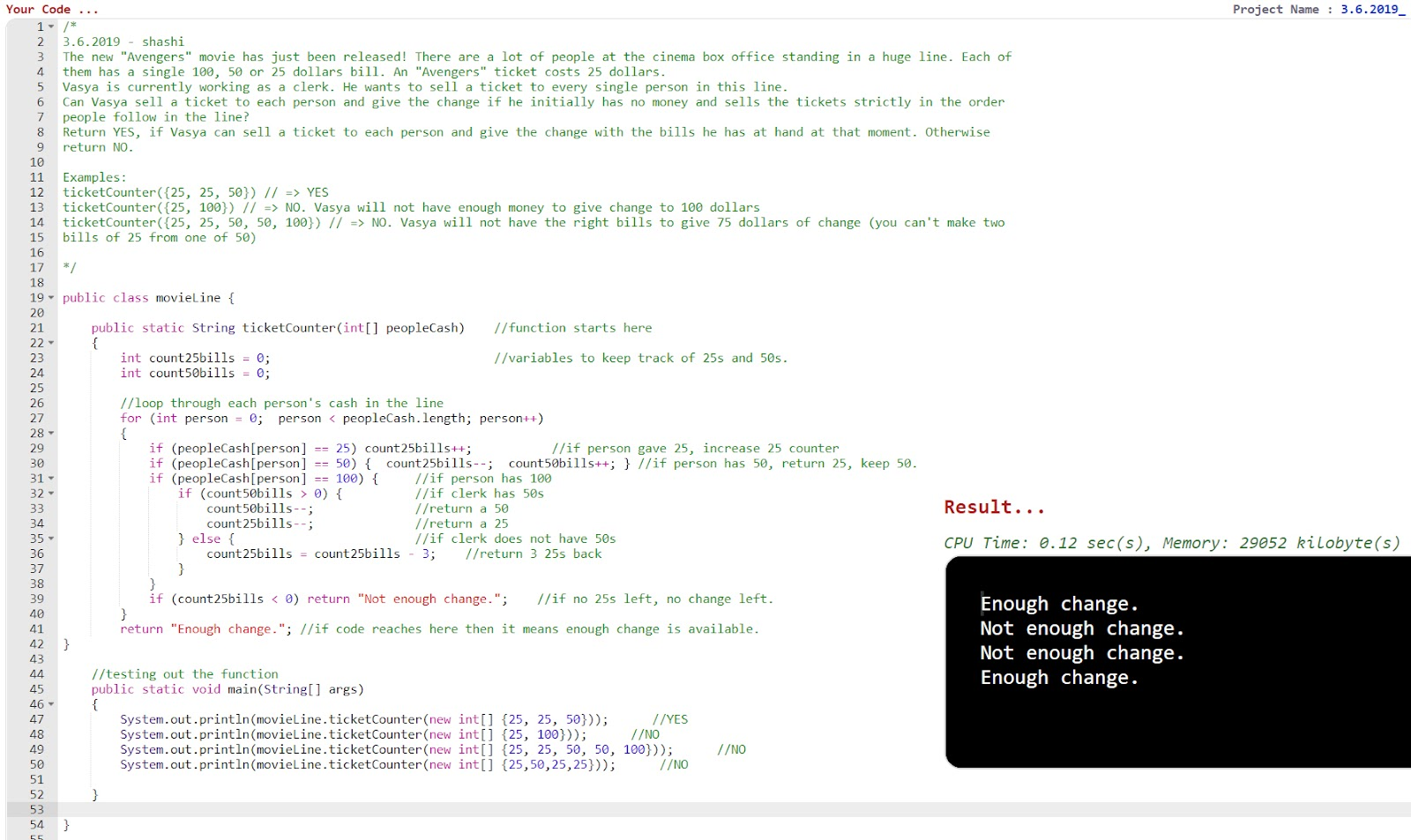### 3.7.2019 | Town’s population calculator depending on growth percentage.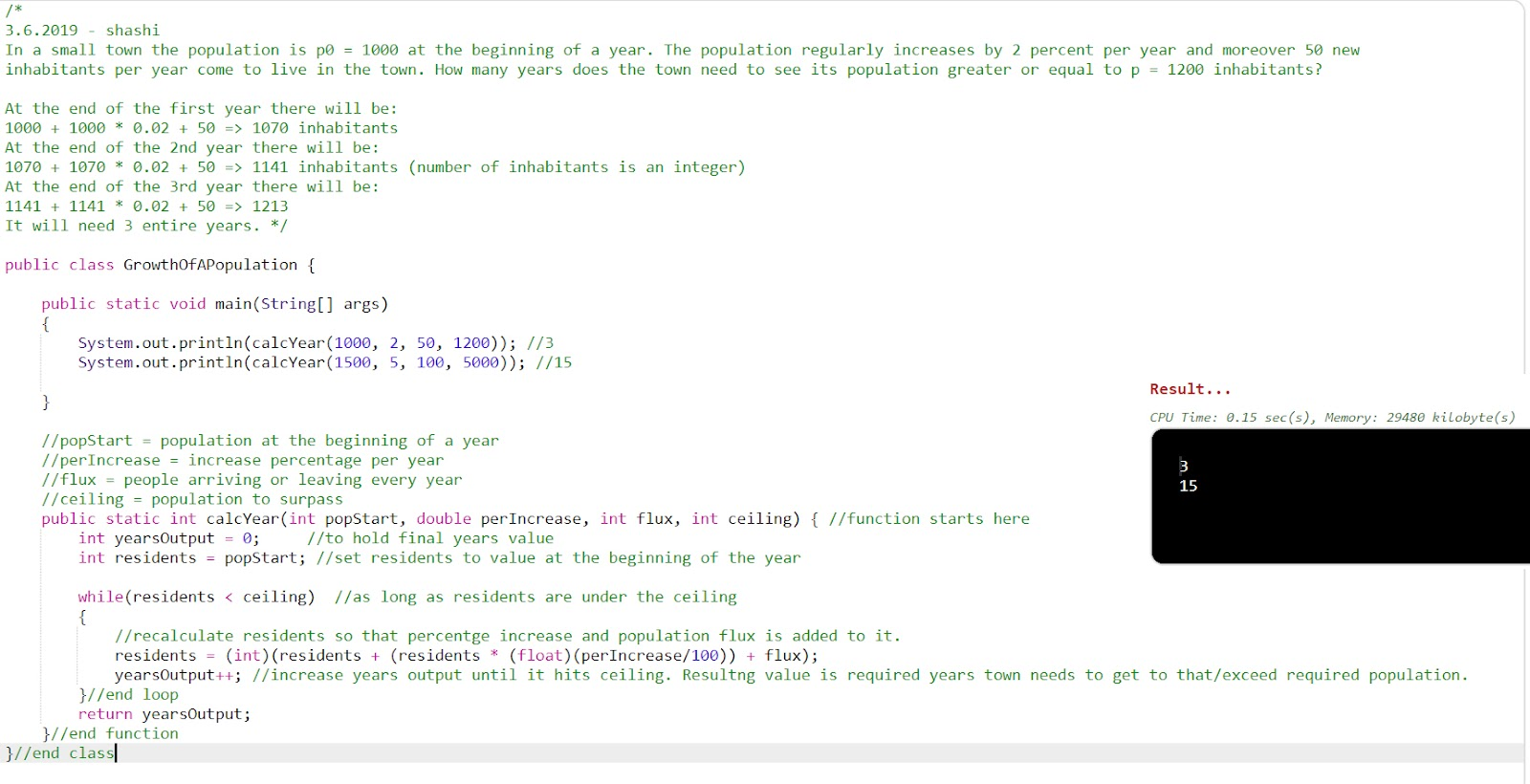### 3.8.2019 | Accept String array of words and format a sentence from them.

 3.8.2019 - shashi
Complete the method so that it formats the words into a single comma separated value.The last word should be separated by the word 'and' instead of a comma. The method takes in an array of strings and returns a single formatted string. Empty string values should be ignored. Empty arrays or null/nil values being passed into themethod should result in an empty string being returned.

formatWords(['ninja', 'samurai', 'ronin']) // should return "ninja, samurai and ronin"
formatWords(['ninja', '', 'ronin']) // should return "ninja and ronin"
formatWords([]) // should return ""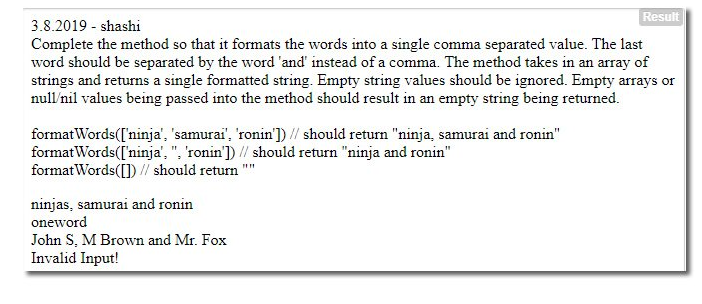### 3.9.2019 - Morse Code Decoder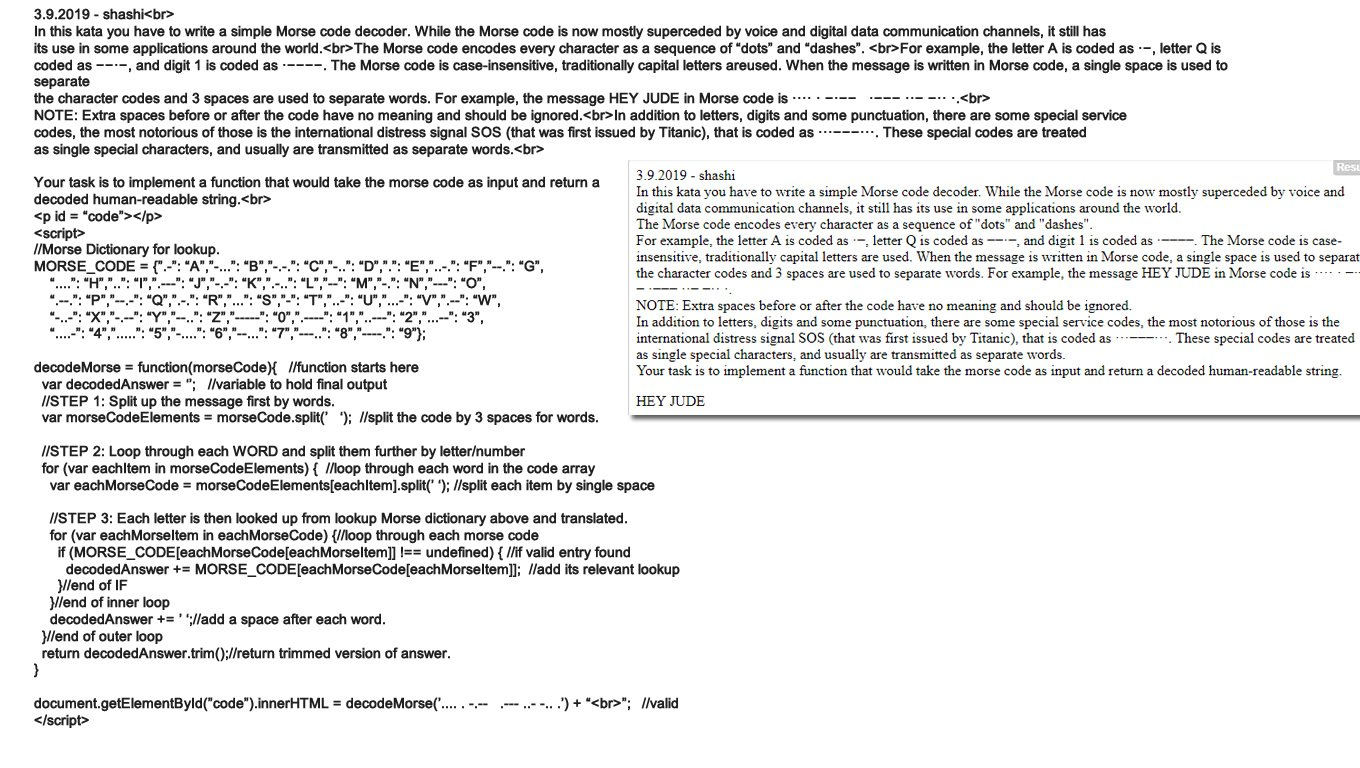### 3.10.2019 - Add two numbers without using the + operator (Bitwise operators)

 3.10.2019 - shashi
Create an addition function that does not utilize the + or - operators.
Anti-cheat tests: You may not use any of these symbols: +-[].'"`

3.10.2019 - shashi

Create an addition function that does not utilize the + or - operators.

Anti-cheat tests: You may not use any of these symbols: +-[].'"`

23

14

### 3.11.2019 - Cipher/Encryption program that converts first letter to ASCII and swaps 2nd and last letter.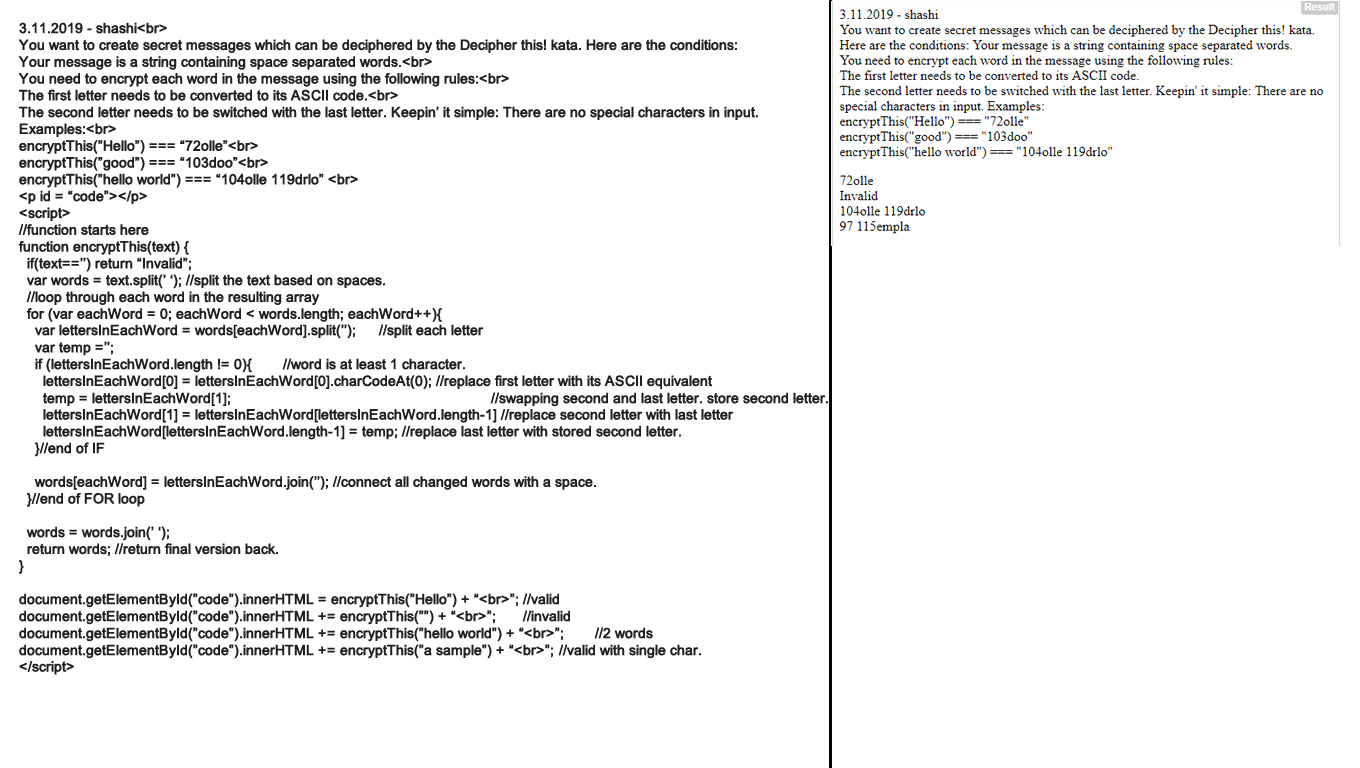### 3.12.2019 - Accept a number and return a String separated/grouped by appearance of even/odd digits.### 3.13.2019 - Stack based post fix notation processor.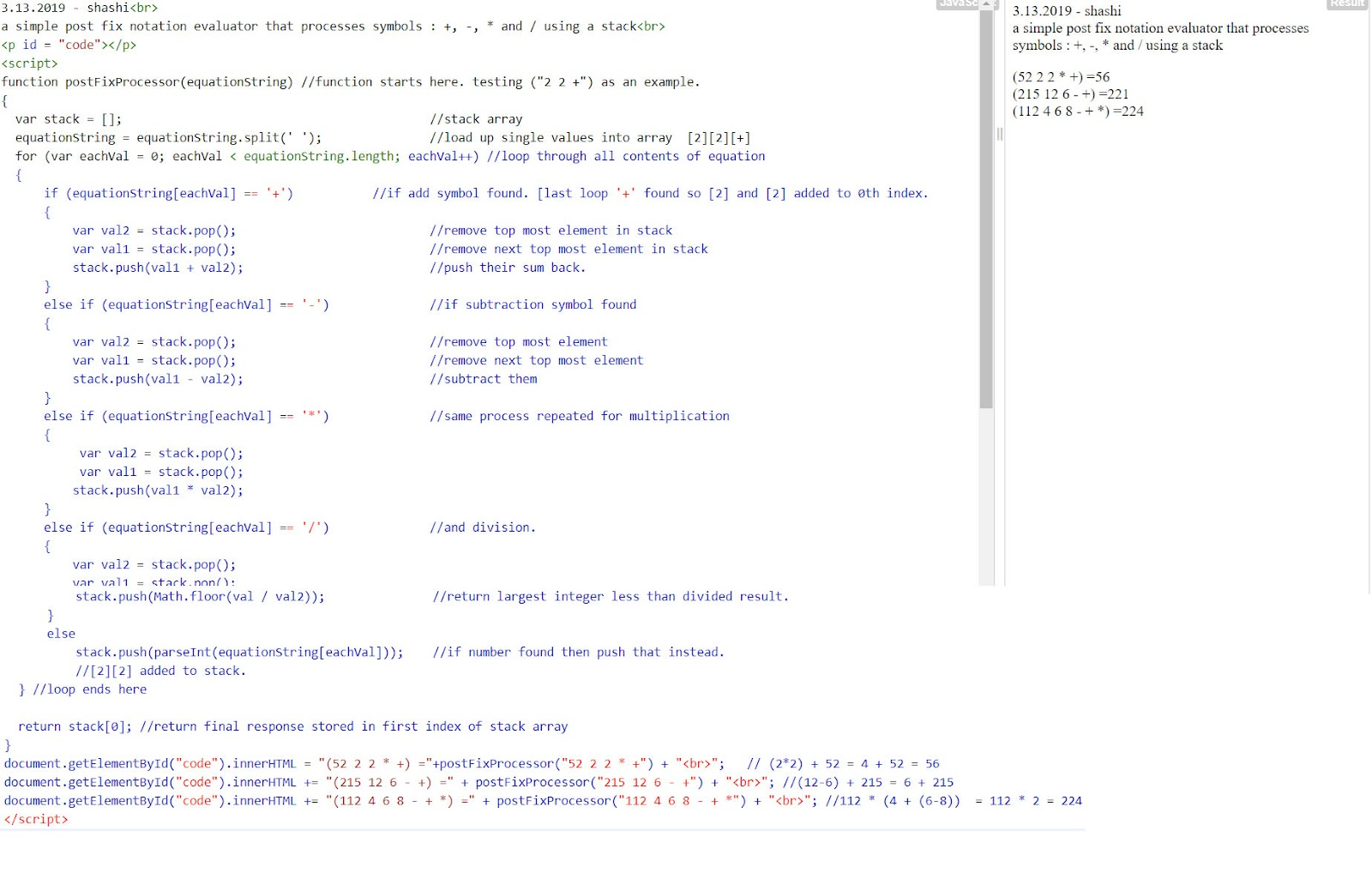### 3.14.2019 | Accept text and format it to specified width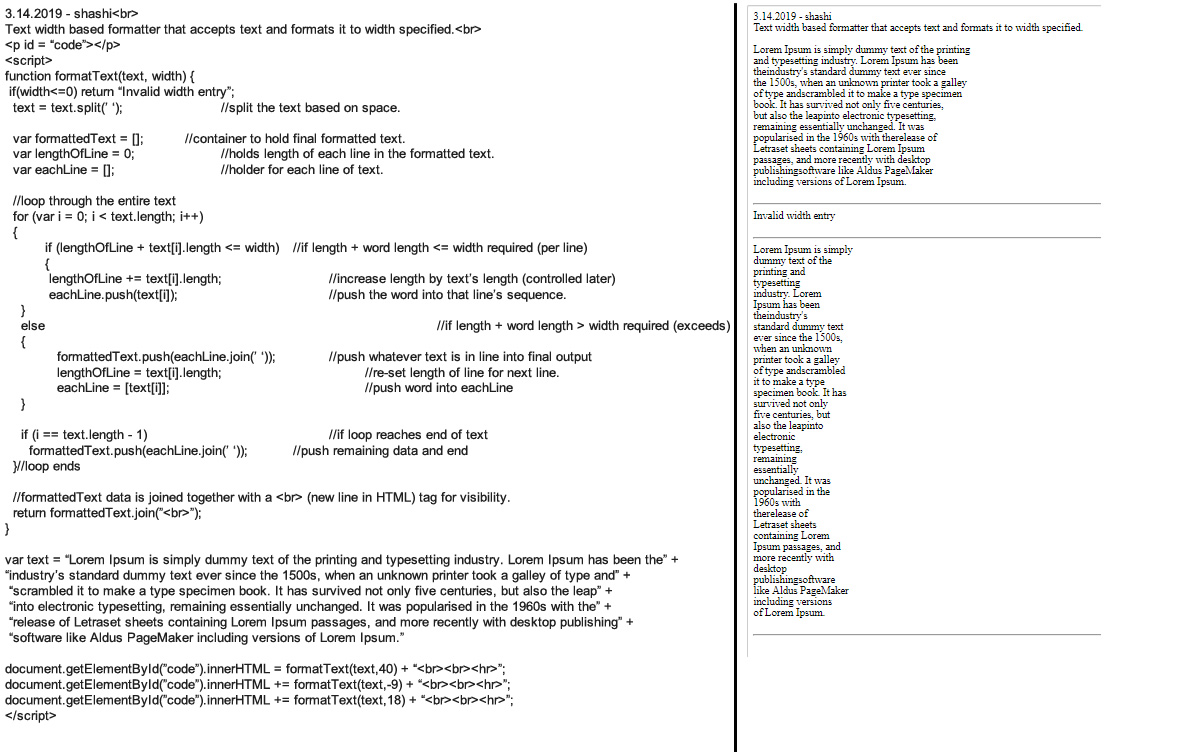### 3.15.2019 | Accept String and create an extended, increasing version of itself.### 3.16.2019 | Accept number and return number of times it need to multiply within for a single digit to result.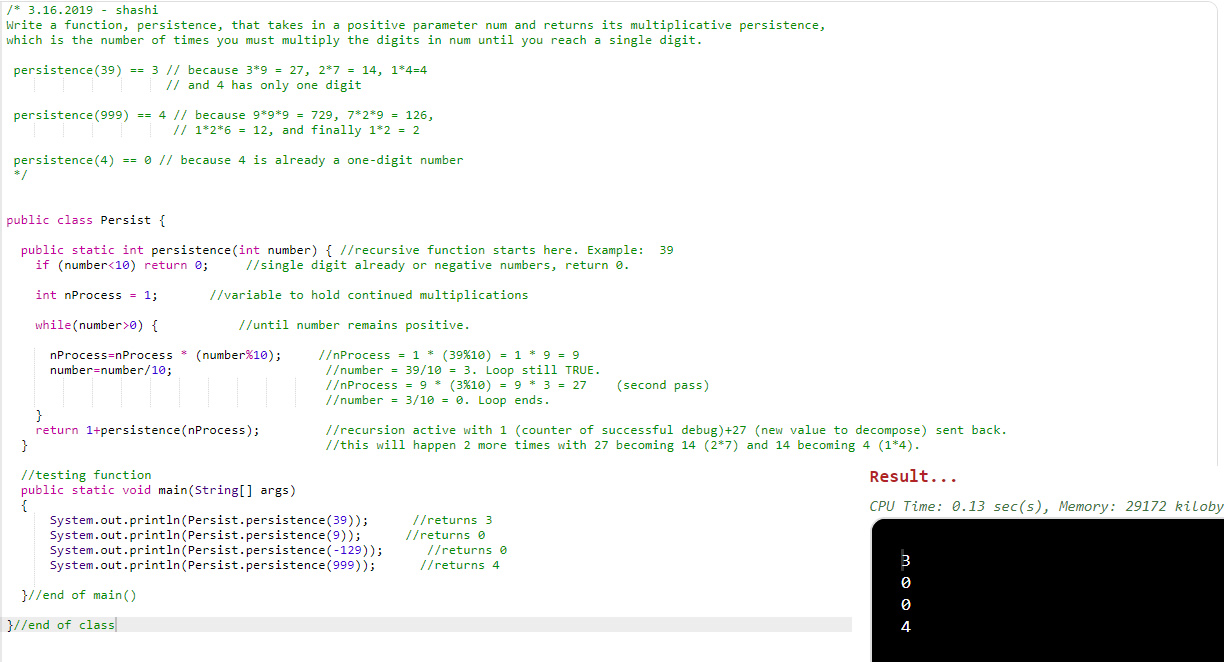### 3.18.2019 | Check if a readable string exists in a scrambled string pattern.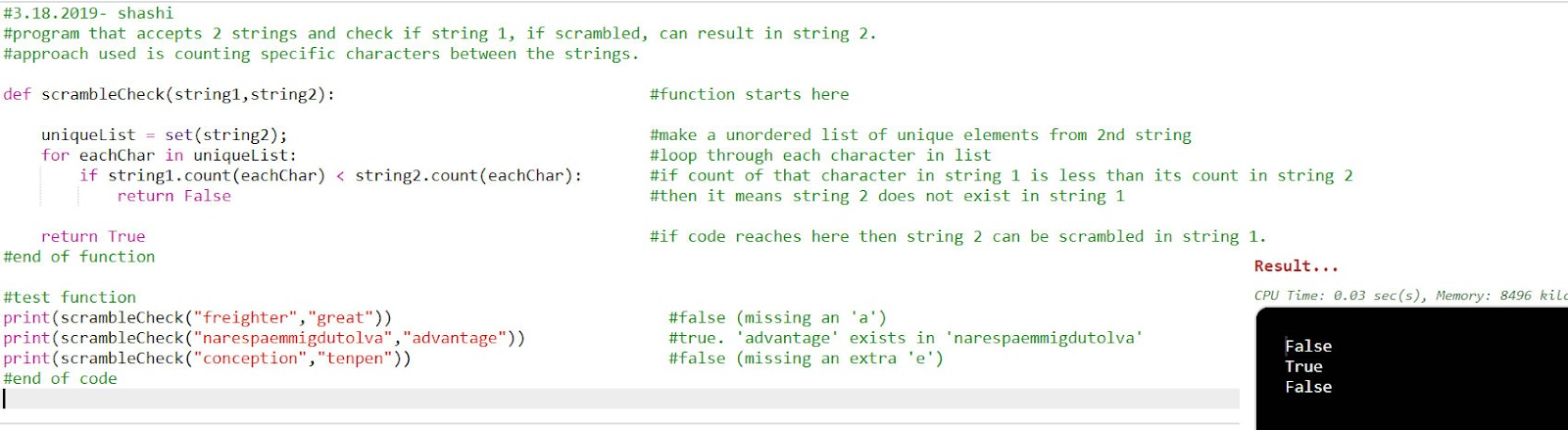### 3.19.2019 | Accept mirror image of a clock time and return the actual time.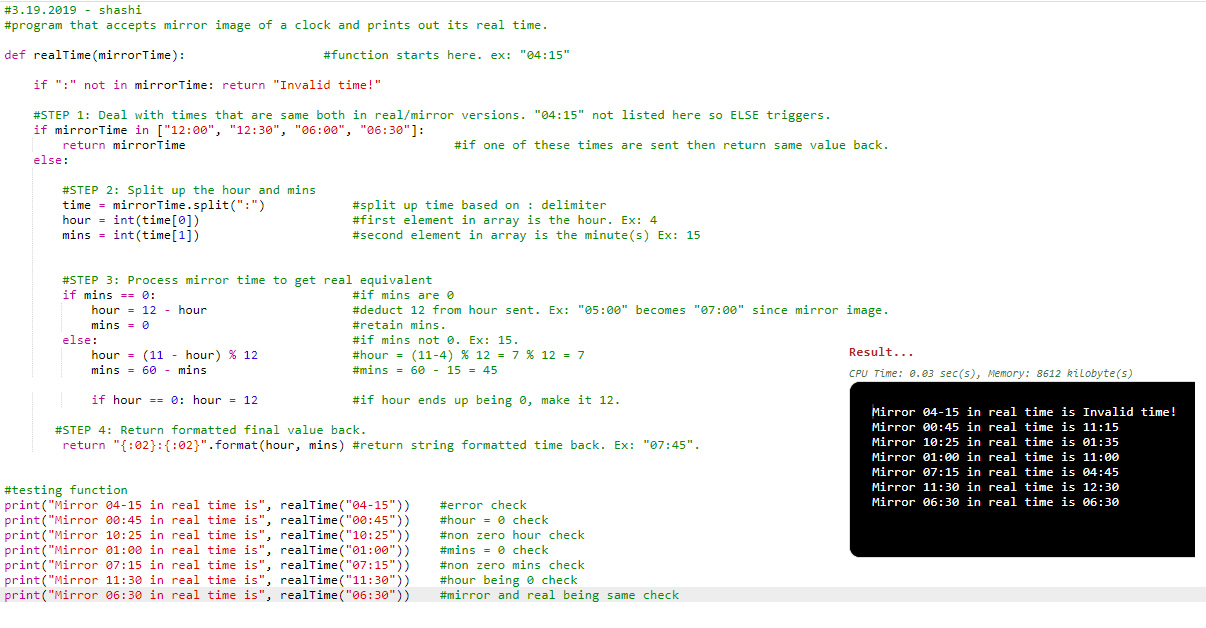### 3.20.2019 | Greed game dice roll calculator

3.19.2019 - shashi<br>

Greed is a dice game played with five six-sided dice. Your mission, should you choose to accept it, is to score a throw according to these rules. You will always be given an array with five six-sided dice values.

<br><br>

Three 1's => 1000 points<br>

Three 6's =>  600 points<br>

Three 5's =>  500 points<br>

Three 4's =>  400 points<br>

Three 3's =>  300 points<br>

Three 2's =>  200 points<br>

One   1   =>  100 points<br>

One   5   =>   50 point<br>

<br>

A single die can only be counted once in each roll. For example, a "5" can only count as

part of a triplet (contributing to the 500 points) or as a single 50 points, but not both

in the same roll.

<br><br>

Example scoring

<br><br>

Throw            &nbsp;&nbsp;&nbsp;&nbsp;Score<br>

---------   &nbsp;&nbsp;&nbsp;&nbsp;   ------------------<br>

5 1 3 4 1  &nbsp;&nbsp;&nbsp;&nbsp;  50 + 2 * 100 = 250<br>

1 1 1 3 1 &nbsp;&nbsp;&nbsp;&nbsp;   1000 + 100 = 1100<br>

2 4 4 5 4  &nbsp;&nbsp;&nbsp;&nbsp;  400 + 50 = 450<br><br>

.

<p id = "code"></p>

<script>

function dieScore( diceRoll ) {

var oneCount=0,

twoCount=0,

threeCount =0;

fourCount=0,

fiveCount=0,

sixCount=0;

var loopCounter = 0;

//STEP 1: Count how many of each possible value exist in die roll.

while (loopCounter < 5) {

if (diceRoll[loopCounter] == 1) { oneCount++; }

if (diceRoll[loopCounter] == 2) { twoCount++; }

if (diceRoll[loopCounter] == 3) { threeCount++; }

if (diceRoll[loopCounter] == 4) { fourCount++; }

if (diceRoll[loopCounter] == 5) { fiveCount++; }

if (diceRoll[loopCounter] == 6) { sixCount++; }

loopCounter++;

}

//STEP 2: Calculate the score based on counts

var result = 0;

if (oneCount > 2) { result += 1000; oneCount = oneCount-3;}

if (twoCount > 2) { result += 200; }

if (threeCount > 2) { result += 300; }

if (fourCount > 2) { result += 400; }

if (fiveCount > 2) { result += 500; fiveCount = fiveCount - 3;}

if (sixCount > 2) { result += 600; }

//additional points for 1s and 5s

result = result +(oneCount * 100);

result = result +(fiveCount * 50);

return result; //final output sent back.

}

//testing function

document.getElementById("code").innerHTML = dieScore( [5, 1, 3, 4, 1]) + "<br>"

document.getElementById("code").innerHTML += dieScore( [4, 4, 4, 3, 3] ) + "<br>"

document.getElementById("code").innerHTML += dieScore( [1, 6, 6, 6, 5] ) + "<br>"

document.getElementById("code").innerHTML += dieScore( [1, 1, 1, 3, 1] ) + "<br>" </script>

### 3.21.2019 | Pizza order rewarding system calculator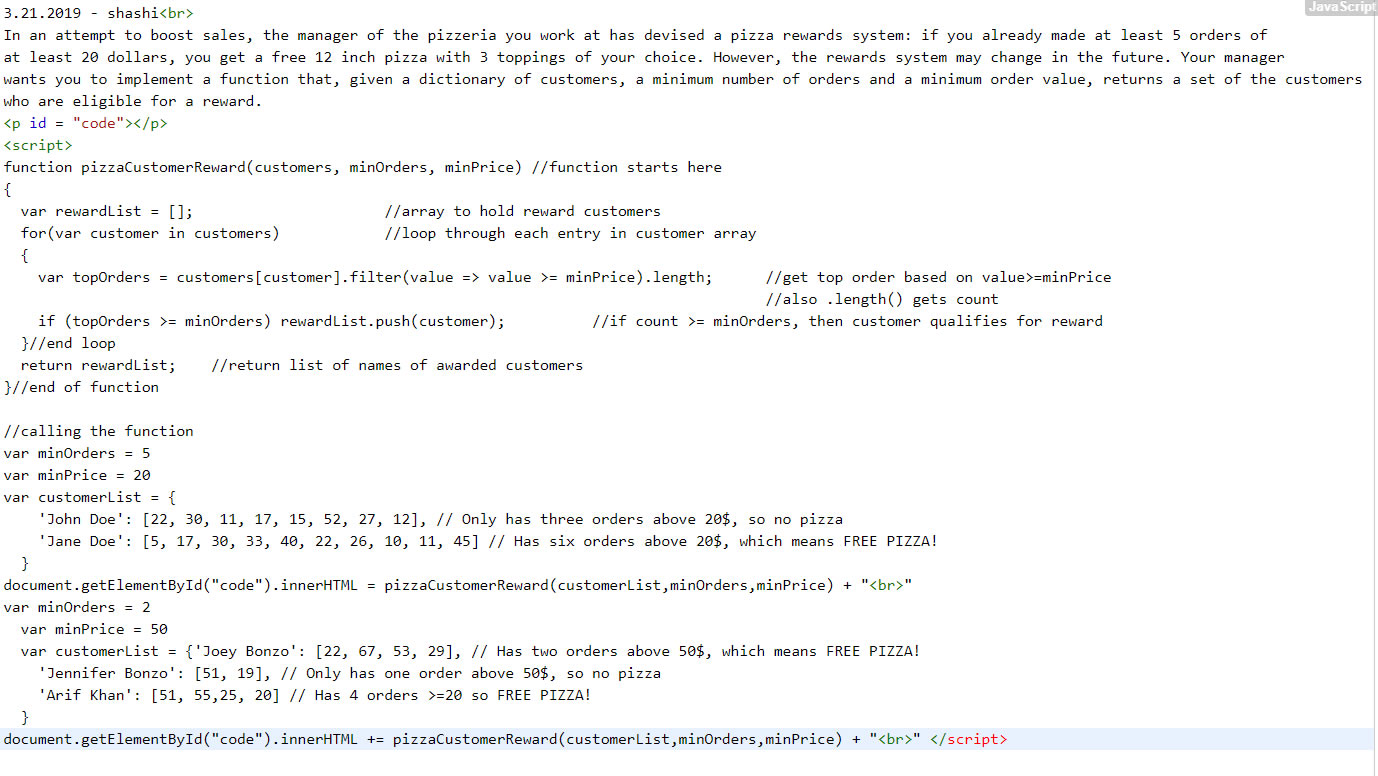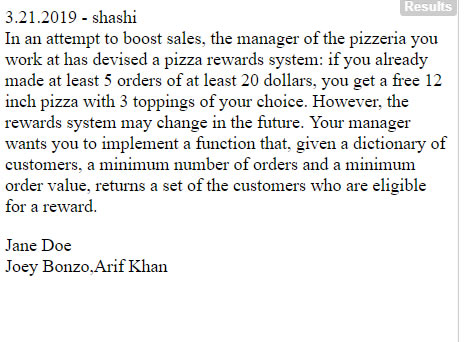### 3.22.2019 | Simple sentence reverser in Java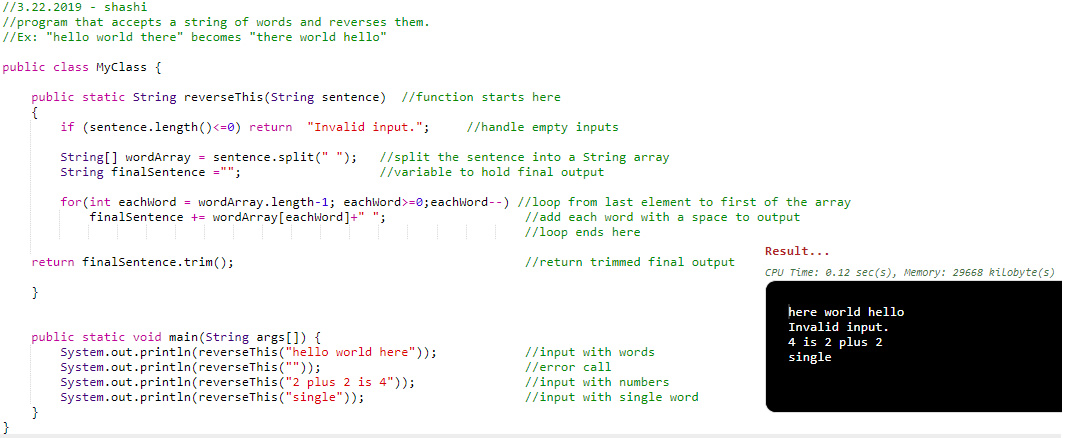### 3.23.2019 | Accept string and treat # symbol as a backspace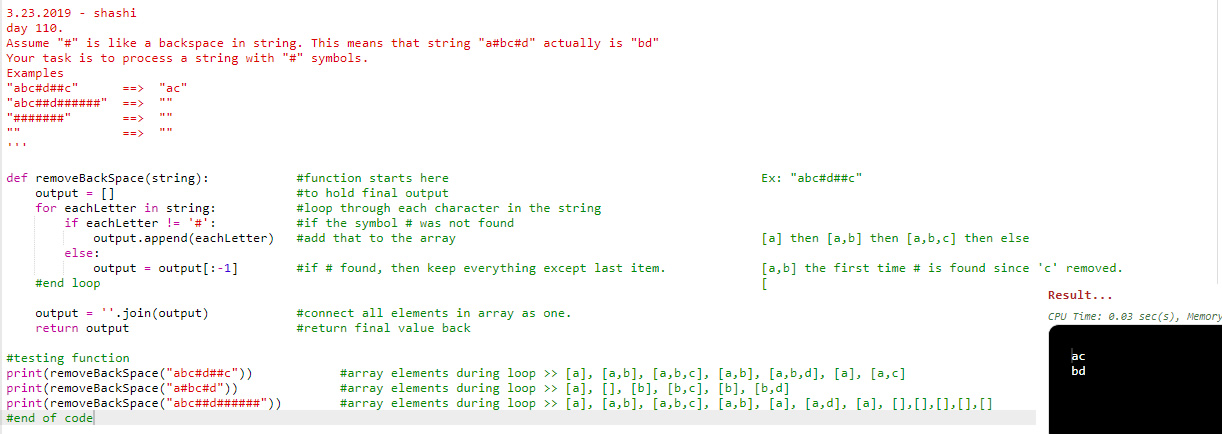### 3.24.2019 | Check if a number can be produced by adding any 2 numbers in array.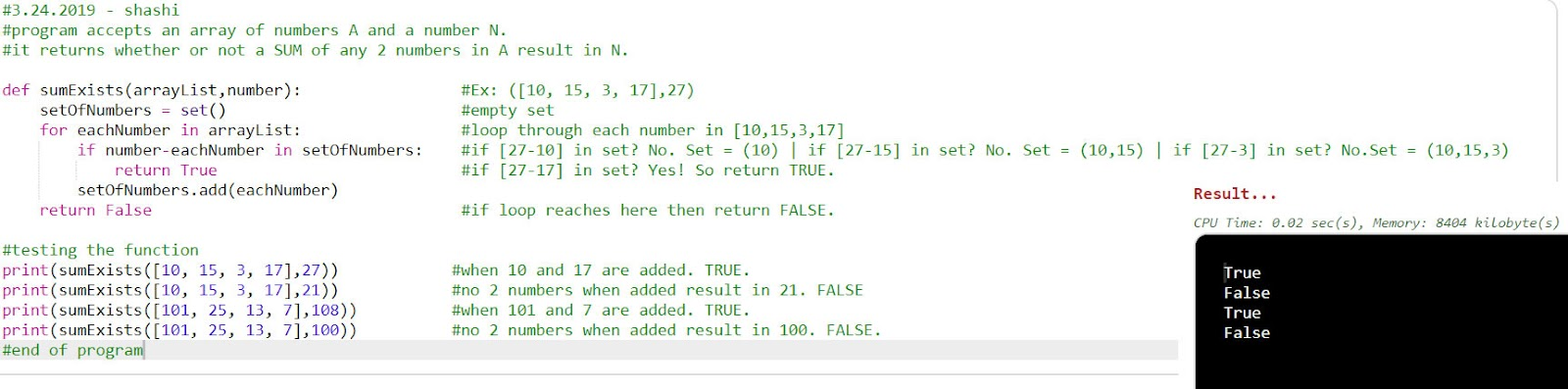### 3.25.2019 | Bowling pin processor that removes pins based on numbers.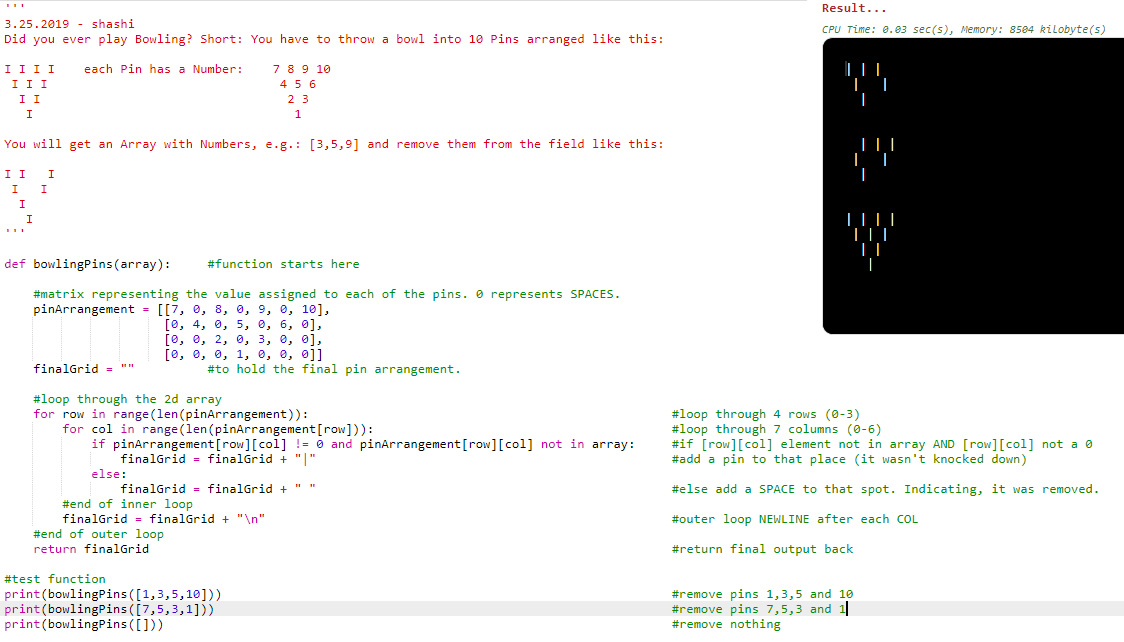### 3.26.2019 | Program accepts truck driver schedule and prints whether or not overtime is reached.

3.26.2019 - shashi<br>

Driving can be really stressfull and weary activity especially if you are driving a big truck. Your task is to write

function which return true if driver exceed daily limit,false otherwise. The only limitation is that driver cant drive in

total more than 9 hours in 24 hours span.<br>

Function input:<br>

//There will be only hours from same day,only 3 types of activites “Drive”,”Rest”,”Work”<br>

//Limit only applies to “Drive” activity<br>

//We assume that before and after described activities driver was resting.<br>

var dailyShedule1 = [ [”7:00-10:30”,”Drive”],<br>

[”10:30-10:45”,”Rest”],<br>

[”10:45-11:45”,”Drive”],<br>

[”11:45-12:15”,”Rest”],<br>

[”12:15-16:45”,”Drive”],<br>

[”16:45-20:15”,”Work”]]; <br>

//-> should return false,9 hours of driving in total. <br>

<p id = “code”></p>

<script>

function overloadCheck(driverSchedule)

{

var totalHoursWorked = 0;

for (var eachSchedule = 0 ; eachSchedule < driverSchedule.length; eachSchedule++)

{

var eachBlock = driverSchedule[eachSchedule];            //load timeslot and activity.

var timeSlot = eachBlock;                                            //load just the timeslot

var activity = eachBlock;                                            //load just the activity

if (activity === “Drive”)                                                     //check if the status is “Drive”

{

var start = timeSlot.match(/[\d]+[:][\d]+/g);            //do a global search for digits matching “digits”+”:”+”digits” pattern in first index element

var finish = timeSlot.match(/[\d]+[:][\d]+/g);            //do a global search for digits matching “digits”+”:”+”digits” pattern in second index element

var startTime = parseInt(start.split(’:’).join(’’));     //get rid of “:” and join the digits.

var finishTime = parseInt(finish.split(’:’).join(’’));    //get rid of “:” and join the digits.

//add subtotal of hours by subtracting finish time - start time.

totalHoursWorked = totalHoursWorked + (finishTime - startTime);

}//end of if

}    //end of loop

if(totalHoursWorked<=900) return “Good to go!”;

else return “Tired! Stop driving!”;

}

//calling the function

var dailyShedule1 = [[”7:00-10:30”,”Drive”],

[”10:30-10:45”,”Rest”],

[”10:45-11:45”,”Drive”],

[”11:45-12:15”,”Rest”],

[”12:15-18:45”,”Drive”],

[”18:45-20:15”,”Work”]];

document.getElementById(”code”).innerHTML = overloadCheck(dailyShedule1) + “<br>”

var dailyShedule2 = [[”5:15-10:15”,”Work”],

[”10:00-19:00”,”Drive”]];

document.getElementById(”code”).innerHTML += overloadCheck(dailyShedule2) + “<br>” </script>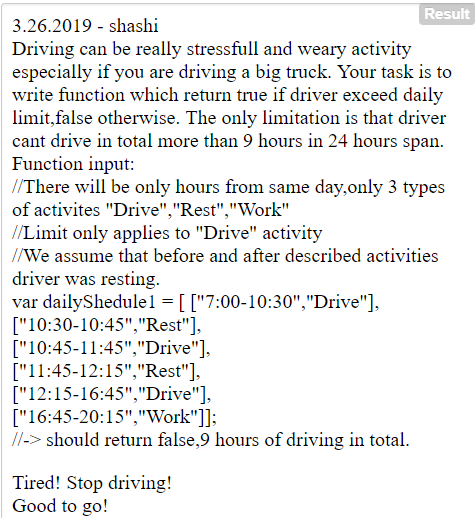### 3.27.2019 | Accept a number, convert to Binary and swap its even/odd bits.

<!–– STEP 1: Set up the HTML. This includes textbox, button and labels needed for output––>

<font face="verdana">

3.28.2019 - shashi (day 114)<br><br>

Program accepts a number in decimal and swaps its bits and returns swapped value equivalent.<br><br>

Enter a number : <input type="text" style="font-size:16px;" size="5" id ="inputNumberID">

<input type="button" onclick="swapBits()" class="button" value="Swap!"><br>    <!––function connected to button.––>

Before Swap :<label id ="before"></label> <br>

After Swap :<label id ="after"></label> <br><hr>

Converted  :<label id="output"></label>

<hr>

</font>

<!–– STEP 2: Write the function that does the swap and connect it to the button click.––>

<script type="text/javascript">

function swapBits() //function starts here

{

var inputNumber = document.getElementById("inputNumberID").value;    //get the value entered in text input on form

var convertedNumber=0;                                                                                            //to hold converted number

var beforeConversion = (+inputNumber).toString(2);                                    //convert original number to Base 2 equivalent

beforeConversion = "00000000".substr(beforeConversion.length) + beforeConversion;    //pad it with leading 0s for 8-bit

document.getElementById("before").innerHTML = beforeConversion;            //print it on the HTML page

//the actual conversion where even bits are shifted right by 1 bit and odd bits are shifted left.

convertedNumber = (((inputNumber & 0xAAAAAAAA) >> 1) | ((inputNumber & 0x55555555) << 1))

//convert new number to Base 2

var afterConversion = (+convertedNumber).toString(2);

afterConversion = "00000000".substr(afterConversion.length) + afterConversion;    //pad it with leading 0s.

document.getElementById("after").innerHTML = afterConversion            //print the converted Base 2 equivalent

document.getElementById("output").innerHTML = convertedNumber;    //print new converted/swapped Base 10 equivalent.

}//end of function

</script>

<!–– STEP 3: (OPTIONAL) Styling of the Swap button (or any other button used on this form)––>

<style>

.button {

background-color: #1c627c;

border: none;

color: white;

padding: 10px 10px;

text-align: center;

text-decoration: none;

display: inline-block;

font-size: 16px;

margin: 4px 2px;

cursor: pointer;

}

</style>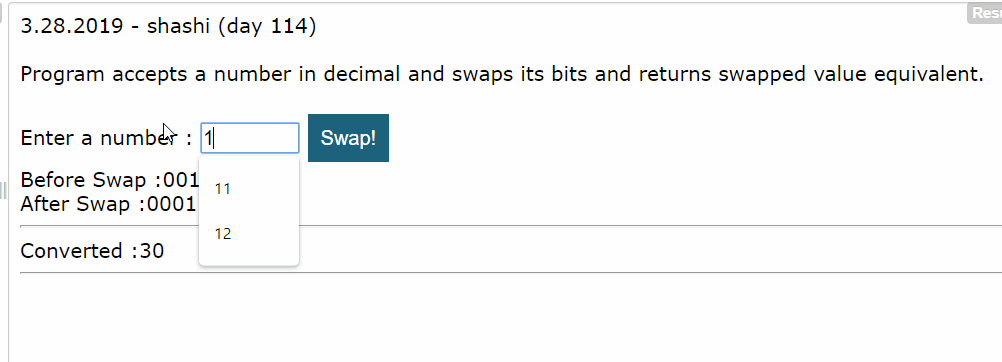### 3.28.2019 | Accept score (0-100) and project completion count to print a final grade.